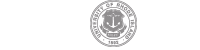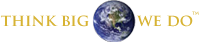# Department of Physics

East Hall, 2 Lippitt Road, Kingston, RI 02881

physics@etal.uri.edu401-874-2104## PHY 510: Mathematical Methods of Physics I

### Course Information

###### Please note that exact details of the course can vary

Professor:Dr.Peter Nightingale

Semester: Fall of odd years
Credits: 3
Prerequisites: permission of chairperson

Catalog Description: Topics designed to include applications in physics. Vector and tensor analysis; linear algebra; coordinate systems. Determinants, matrices; introductory group theory. Infinite series, complex analysis,analytic properties, conformal mapping, calculus of residues. Fourier analysis and Laplace transforms.

Course Goals & Outcomes

Use their understanding of linear algebra to calculate eigenvalues and eigenvectors, to determine coordinate transformations, to solve sets of linear equations with a variety of methods, to decompose matrices into useful products and demonstrate the ability to select the best method for the problem at hand and to be able to derive the properties of Hermitian matrices.

Use their understanding of probability to calculate uncertainties, to model systems using Markov chains and to perform mathematical calculations using Monte Carlo techniques

Course Description

Topics designed to include applications in physics: linear algebra; determinants, matrices, eigenvalues; properties of finite and infinite bases; basics of numerical linear algebra; probability and statistics; Monte Carlo methods.

The course will be based on the i) lectures material, and ii) textbook by I. J. Matthews and R. L. Walker, Mathematical Methods of Physics.

Grading will be based on project(s), midterm and final exams. Exams will test the understanding of theoretical methods and their applications

Students will submit projects in electronic form .

Topics covered in this course include:

• Linear vector spaces and operators
• Linear vector spaces and operators
• Matrices and coordinate transformations
• Eigenvalues, variational principles
•  Infinite spaces
• Properties of bases and examples
• Determinants and Gaussian elimination
• LU decomposition, range, null-space, norms
• Properties of Hermitian matrices
• geometric and algebraic multiplicity
• Singular value decomposition
• Review of probability theory and statistics
•  Time series and correlations
• Markov chains and random deviates
• Monte Carlo Integration and the Metropolis algorithm